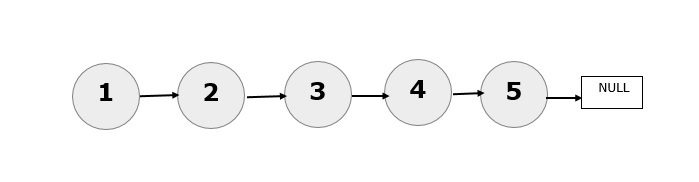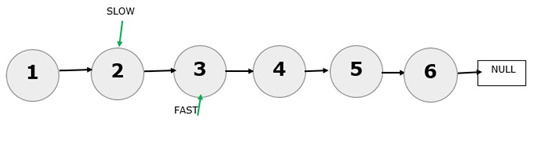# Python Program To Get The Middle Element Of A Linked List In A Single Iteration

Linked list is used for storing the data in non-contiguous memory locations. The nodes containing the data items are linked using pointers. Each node consists of two fields. The first field is used for storing the data and the second field contains the link to the next node.

### Brute Force Technique

To find the middle element of a linked list, the brute force technique is to find out the length of the linked list by iterating the entire linked list till NULL is encountered and then the length is divided by 2 to get the index of the middle element. After getting the index of the middle element, iterate the linked list again from the head and stop when the required index is reached. The data item at that index gives the middle element.

• Take a variable called “temp” pointing to the HEAD and initialize “len” to 0

• Iterate the linked list using temp until NULL is reached and increment “len” by 1 at every node.

• After getting the length of the linked list, initialize the temp to HEAD again. Iterate the linked list until len//2.

## Using Slow And Fast Pointers (Single Iteration)

We will use two pointers to traverse the linked list. One is called the “slow pointer” and the other is called the “fast pointer”.

Fast pointer will be moving with double the speed of the slow pointer.

When the fast pointer reaches the end of the linked list, The slow pointer will be at the middle node.

Hence, we can directly print the contents of the middle node.

### Example

Consider the below linked list. The middle element is 3.The fast pointer has reached the last node in the linked list and now the slow pointer is pointing to the node 3. Hence, 3 is the middle element of the given linked list. Now, consider 6 nodes.### Example

The fast pointer has reached NULL and the slow pointer is pointing towards the 4th node. Hence, the middle element is 4.

### Algorithm

• Make the “slow” and “fast” point to the HEAD of the linked list.

• Increment fast pointer by two and the slow pointer by one until that fast pointer and fast.next are not equal to NULL

• Print the value at the slow pointer.

• The time complexity will be O(n).

class Node:
def __init__(self, val):
self.val = val
self.next = None
def __init__(self):

def insert_at_the_beginning(self, newVal):
newNode = Node(newVal)
def print_middle_element(self):
while fast is not None and fast.next is not None:
slow=slow.next      #slow pointer moves one node
fast=fast.next.next  #fast pointer moves two nodes
print("\n\nthe middle element is ",slow.val)
def Print_the_LL(self):
if(temp != None):
print("The linked list elements are:", end=" ")
while (temp != None):
print(temp.val, end=" ")
temp = temp.next
else:
print("The list is empty.")
newList.insert_at_the_beginning(5)
newList.insert_at_the_beginning(4)
newList.insert_at_the_beginning(3)
newList.insert_at_the_beginning(2)
newList.insert_at_the_beginning(1)
newList.Print_the_LL()
newList.print_middle_element()


### Output

The linked list elements are: 1 2 3 4 5

the middle element is  3


Updated on: 24-Apr-2023

688 Views